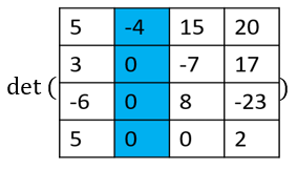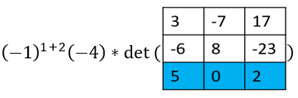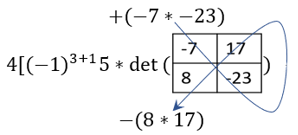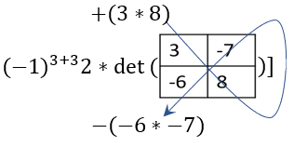# Determinant

The determinant of an n x n square matrix A, denoted |A| or det (A), in one of its simpler definitions, is a value that can be calculated from a square matrix. The determinant of a matrix has various applications in the field of mathematics including use with systems of linear equations, finding the inverse of a matrix, and calculus. The focus of this article is the computation of the determinant. Refer to the matrix notation page if necessary for a reminder on some of the notation used below. There are a number of methods for calculating the determinant of a matrix, some of which are detailed below.

### Determinant of a 2 × 2 matrix

Given a 2 × 2 matrix, below is one way to remember the formula for the determinant. Refer to the figure below. You can draw a fish starting from the top left entry a. When going down from left to right, you multiply the terms a and d, and add the product. When going down from right to left you multiply the terms b and c and subtract the product.

### Geometric meaning of a determinant

is a number that represents the "signed volume" of the parallelepiped (the higher dimensional version of parallelograms) spanned by its column or row vectors. The term "signed volume" indicates that negative volume is possible in cases when the parallelepiped is turned "inside out" in some sense. For example, if we switch 2 vectors of the parallelepiped, we are essentially pushing 2 of the sides past each other until the interior of the parallelepiped faces outwards and the former exterior now faces inwards. In 3D, a parallelepiped spanned by vectors v1, v2, and v3 looks like a slanted box:

### Cofactor expansion

Cofactor expansion, sometimes called the Laplace expansion, gives us a formula that can be used to find the determinant of a matrix A from the determinants of its submatrices.

We define the i-jth submatrix of A, denoted Aij (not to be confused with aij, the entry in the ith row and jth column of A), to be the matrix left over when we delete the ith row and jth column of A. For example if i = 2 and j = 4, then the 2nd row and 4th columns indicated in blue are removed from the matrix A below:

resulting in matrix Aij:

The i-jth cofactor, denoted Ci, j, is defined as. This definition gives us the formula below for the determinant of a matrix A:Be careful not to confuse Aij, the i-jth submatrix, with aij, the scalar entry in the ith row and the jth column of A. This formula is called the "cofactor expansion across the ith row. Notice that in this formula, j is changing but i remains fixed. This represents moving across the ith row and adding and subtracting the current entry aij times the current cofactor Cij in an alternating pattern.

Similarly, the cofactor expansion formula down the jth column isExample

When performing cofactor expansion, it is very useful to expand along rows or columns that have man 0's since if aij = 0, we won't have to calculatebecause it will just be multiplied by 0. This greatly reduces the number of steps needed. Below, blue represents the column or row we are expanding along.==+=+==Given that the matrix is square, cofactor expansion can be used to find the determinants of larger square matrices as shown above. The bigger the matrix however, the more cumbersome the computation of the determinant.